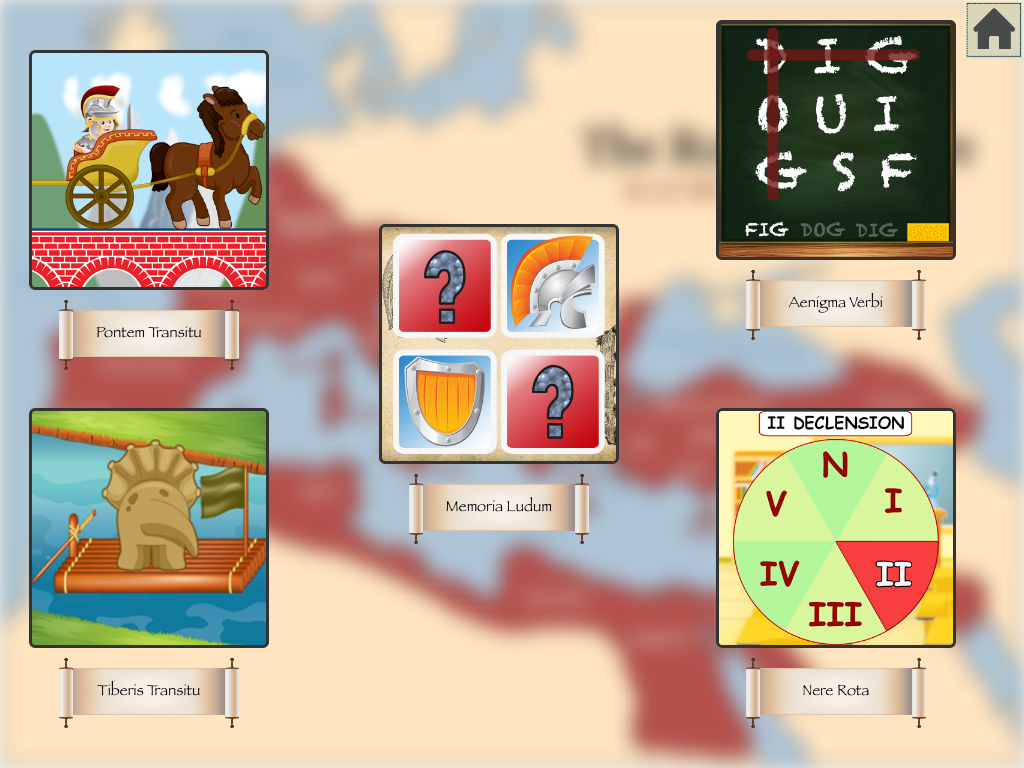## Learn Latin with @Reks iOS App!Lingua Latina Nouns iOS app created by @Reks helps students learn Latin nouns, practice them through five different games, and finally gives students a chance to take a test and see how much knowledge they acquired. A student chooses whether he wishes to learn or practice the meaning of nouns or grammar. All these and many […]

## Lingua Latina Nouns iOS AppThis iOS app helps students learn Latin nouns, practice them through five different games, and finally gives students a chance to take a test and see how much knowledge they acquired. A student chooses whether he wishes to learn or practice the meaning of nouns or grammar. All these and many other options are available in […]

## 2nd Grade Printable Math Quiz WorksheetsYou can download a number of math practice worksheets designed for Grade 2  Elementary School. The practice worksheets are based on Math Essentials textbook and math common core standards. Unit 1 covers the following concepts: add and subtract numbers up to 20 add three or four numbers find partners and totals write equations for math mountains tell […]

## 5th Grade Printable Math Quiz SheetsThis page offers a number of math worksheets for Grade 5 based on Math Essentials math textbook and common core standards. Unit 1 covers the following topics: – fractions – simplify fractions – addition and subtraction of fractions – word problems with fractions Tap/click the following links to print math tests from Unit 1: 1. 5th Grade Unit 1 […]